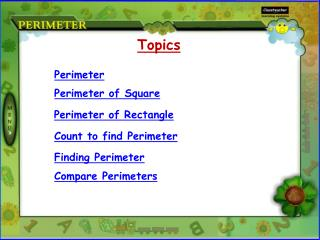DownloadDownload PresentationPerimeter

# Perimeter

Télécharger la présentation## Perimeter

- - - - - - - - - - - - - - - - - - - - - - - - - - - E N D - - - - - - - - - - - - - - - - - - - - - - - - - - -
##### Presentation Transcript

1. Topics Perimeter Perimeter of Square Perimeter of Rectangle Count to find Perimeter Finding Perimeter Compare Perimeters

2. PERIMETER Perimeter is the distance around the outside edge of a flat object.

3. Length Length Length Length Perimeter of Square Example:Find the perimeter of square. Perimeter of Square (P) = length + length + length + length 6 cm P = 4 × Length P = 4 × 6 cm P = 24 cm P = l + l+ l + l P = 4l P= 4 × Length 6 cm 6 cm 6cm

4. length width width length Perimeter of Rectangle Example:Find the perimeter of rectangle. Perimeter of Rectangle (P) = length + width + length + width 8 cm P = 2 ( Length + Width) P = 2 ( 8 cm + 6 cm ) P = 2 × 14 cm P = 28 cm P = l + w + l + w P = 2l + 2w P = 2 (l + w) 6 cm 6 cm P= 2 ( Length + Width ) 8 cm

5. Count to find Perimeter Count the outer sides to determine the perimeter of shape if length of each side is 1 unit. Count the outer sides to determine the perimeter of shape if length of each side is 1 unit. Perimeter = 12 units Perimeter = 24 units

6. Finding Perimeter 3cm 5cm 4 cm 4 cm Perimeter = 4 cm + 4 cm + 3 cm + 3 cm + 4 cm + 4 cm + 3 cm + 3 cm 3cm 3cm 7cm 7cm 3 cm 3 cm 3cm 3cm Perimeter = 28 cm 3 cm 3 cm 3cm 3cm Perimeter = 3 cm + 3 cm + 3 cm + 3 cm + 3 cm + 3 cm Perimeter = 5 cm + 7 cm + 3 cm + 7 cm 4 cm 4 cm Perimeter = 18 cm Perimeter = 22 cm

7. Figure A Figure B Compare Perimeters Find which of the following figure has greater perimeter if side of each square has length 1 cm? Figure B has greater Perimeter. Perimeter = 12 cm Perimeter = 14 cm